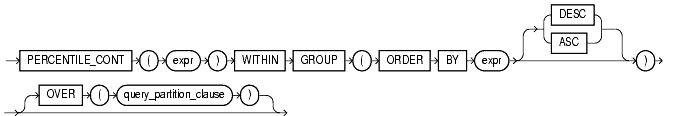Functions, 89 of 166

## PERCENTILE_CONT

#### Syntax

percentile_cont::=Text description of `percentile_cont`

 See Also: "Analytic Functions" for information on syntax, semantics, and restrictions of the `OVER` clause

#### Purpose

The `PERCENTILE_CONT` function is an inverse distribution function that assumes a continuous distribution model. It takes a percentile value and a sort specification, and returns an interpolated value that would fall into that percentile value with respect to the sort specification. Nulls are ignored in the calculation.

The first expr must evaluate to a numeric value between 0 and 1, because it is a percentile value. This expr must be constant within each aggregation group. The `ORDER` `BY` clause takes a single expression that must be a numeric or datetime value, as these are the types over which Oracle can perform interpolation.

The result of `PERCENTILE_CONT` is computed by linear interpolation between values after ordering them. Using the percentile value (P) and the number of rows (N) in the aggregation group, we compute the row number we are interested in after ordering the rows with respect to the sort specification. This row number (RN) is computed according to the formula `RN = (1+ (P*(N-1))`. The final result of the aggregate function is computed by linear interpolation between the values from rows at row numbers `CRN = CEILING(RN)` and `FRN = FLOOR(RN)`.

The final result will be:

```  if (CRN = FRN = RN) then
(value of expression from row at RN)
else
(CRN - RN) * (value of expression for row at FRN) +
(RN - FRN) * (value of expression for row at CRN)

```

You can use the `PERCENTILE_CONT` function as an analytic function. You can specify only the query_partitioning_clause in its OVER clause. It returns, for each row, the value that would fall into the specified percentile among a set of values within each partition.

#### Aggregate Example

The following example computes the median salary in each department.

```SELECT department_id,
PERCENTILE_CONT(0.5) WITHIN GROUP (ORDER BY salary DESC)
"Median cont",
PERCENTILE_DISC(0.5) WITHIN GROUP (ORDER BY salary DESC)
"Median disc"
FROM employees
GROUP BY department_id;

DEPARTMENT_ID Median-cont Median-disc
------------- ----------- -----------
10        4400        4400
20        9500       13000
30        2850        2900
40        6500        6500
50        3100        3100
60        4800        4800
70       10000       10000
80        8800        8800
90       17000       17000
100        8000        8200
110       10150       12000

```

`PERCENTILE_CONT` and `PERCENTILE_DISC` may return different results. `PERCENTILE_CONT` returns a computed result after doing linear interpolation. `PERCENTILE_DISC` simply returns a value from the set of values that are aggregated over. When the percentile value is 0.5, as in this example, `PERCENTILE_CONT` returns the average of the two middle values for groups with even number of elements, whereas `PERCENTILE_DISC` returns the value of the first one among the two middle values. For aggregate groups with an odd number of elements, both functions return the value of the middle element.

#### Analytic Example

In the following example, the median for Department 60 is 4800, which has a corresponding percentile (`Percent_Rank`) of 0.5. None of the salaries in Department 30 have a percentile of 0.5, so the median value must be interpolated between 2900 (percentile 0.4) and 2800 (percentile 0.6), which evaluates to 2850.

```SELECT last_name, salary, department_id,
PERCENTILE_CONT(0.5) WITHIN GROUP (ORDER BY salary DESC)
OVER (PARTITION BY department_id) "Percentile_Cont",
PERCENT_RANK()
OVER (PARTITION BY department_id ORDER BY salary DESC)
"Percent_Rank"
FROM employees WHERE department_id IN (30, 60);

LAST_NAME         SALARY DEPARTMENT_ID Percentile_Cont Percent_Rank
------------- ---------- ------------- --------------- ------------
Raphaely           11000            30            2850            0
Khoo                3100            30            2850           .2
Baida               2900            30            2850           .4
Tobias              2800            30            2850           .6
Himuro              2600            30            2850           .8
Colmenares          2500            30            2850            1
Hunold              9000            60            4800            0
Ernst               6000            60            4800          .25
Austin              4800            60            4800           .5
Pataballa           4800            60            4800           .5
Lorentz             4200            60            4800            1
```